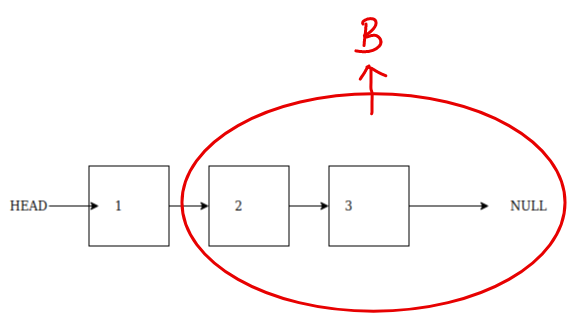# How to print a linked list in reverse ?

## How to print a linked list in reverse?

Printing a linked list in reverse is similar to How to recursively print a linked list ?. If you are not familiar with that problem, we would suggest to go through it first.

Linked List is a linear data structure in which each node points to the next node in the linked list.
Linked List is also a recursive data structure, for example we can say that each node of a linked list points to another linked list as shown in the diagrams below:Linked list thought of normally

We can also think of the above linked list as this:Linked List thought recursively

When we think of the linked list as recursive data structure, as shown in the diagram above. We can say that Node 1 points to another linked list (B).
This observation will help us print the linked list in reverse using recursion.

Therefore, to print this linked list in reverse using recursion, we will write a function which prints the remaining linked list in reverse (using recursion) and then print the current node.

``````// curr is the current node that we want to print
void printLinkedListRecursively(Node* curr) {
if (curr != NULL) {
printLinkedList(curr->next);
cout << curr->data << " ";
}
}

//We will call the above function as
printLinkedList(head);
``````
Scroll to Top

### Full Stack Integrated Bootcamp Free Trial

• Please enter a number from 7000000000 to 9999999999.